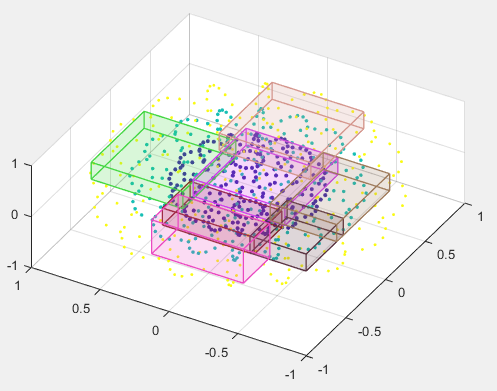# beginDrawingFromPoint

Package: images.roi

Begin drawing ROI from specified point

## Syntax

``beginDrawingFromPoint(ROI,[x y])``
``beginDrawingFromPoint(ROI,[x y z])``
``beginDrawingFromPoint(ROI,[x y z],s)``
``beginDrawingFromPoint(ROI,[x y z],pos)``

## Description

example

``` `beginDrawingFromPoint(ROI,[x y])` enters interactive mode to draw the shape for object `ROI`. The drawing starts at location (x,y) in the axes. This method is intended to be used within the `ButtonDownFcn` callback of an `Image` or `Axes` object.```
``` `beginDrawingFromPoint(ROI,[x y z])` enters interactive mode to draw a cuboidal `ROI` (`images.roi.Cuboid`). The drawing starts at location (x,y,z) in the axes.```
``` `beginDrawingFromPoint(ROI,[x y z],s)` enters interactive mode to draw a cuboidal `ROI` (`images.roi.Cuboid`). The drawing starts at location (x,y,z) in the axes, snapping to the nearest location to the mouse from the `Scatter` object `s`.```
``` `beginDrawingFromPoint(ROI,[x y z],pos)` enters interactive mode to draw a cuboidal `ROI` (`images.roi.Cuboid`). The drawing starts at location (x,y,z) in the axes, snapping to the nearest location to the mouse from the position specified by `pos`. ```

## Examples

collapse all

Create a new script called `sampleDrawLine.m`. Inside the script, copy and paste this code, then save the file.

```hIm = imshow(imread('coins.png')); hIm.ButtonDownFcn = @(~,~) buttonPressedCallback(hIm.Parent); function buttonPressedCallback(hAx) cp = hAx.CurrentPoint; cp = [cp(1,1) cp(1,2)]; obj = images.roi.Line('Parent',hAx,'Color',rand([1,3])); beginDrawingFromPoint(obj,cp); end```

Return to the MATLAB® command window. Run the script by entering the command:

`sampleDrawLine`

The code opens a figure window containing an image of coins. Each time you click the mouse over the figure, the script executes the callback function, `buttonPressedCallback`. The callback function begins drawing a new ROI starting from the pixel you clicked.

In the editor, open a file called `cuboidExample.m`. Copy and paste this code into the file and then save it.

```function cuboidExample [x,y,z] = sphere(16); X = [x(:)*.5 x(:)*.75 x(:)]; Y = [y(:)*.5 y(:)*.75 y(:)]; Z = [z(:)*.5 z(:)*.75 z(:)]; % Specify the size and color of each marker. S = repmat([1 .75 .5]*10,numel(x),1); C = repmat([1 2 3],numel(x),1); % Create a 3-D scatter plot figure hScatter = scatter3(X(:),Y(:),Z(:),S(:),C(:),'filled'); view(-60,60); % Begin drawing cuboids when a scatter % point is clicked hScatter.ButtonDownFcn = @(~,~) buttonPressedCallback(hScatter.Parent); function buttonPressedCallback(hAx) cp = hAx.CurrentPoint; cp = cp(1,1:3); obj = images.roi.Cuboid('Parent',hAx,'Color',rand([1,3])); obj.beginDrawingFromPoint(cp); end end```

Return to the MATLAB command window and run the function by entering the command:

`cuboidExample`

The code opens a figure window containing a scatter plot. Each time you click the mouse over the scatter plot, the function executes the callback function, `buttonPressedCallback`, and draws a new cuboidal ROI at the pixel you clicked.## Input Arguments

collapse all

Region of interest, specified as an ROI object of one of the following types:

Starting point in the axes, specified as a numeric array.

Starting point in 3-D axes, specified as a numeric array.

Scatter plot, specified as a `matlab.graphics.chart.primitive.Scatter` object.

Starting point in 3-D axes, specified as an N-by-3 numeric array. Each row in `pos` represents a 3-D spatial location of a potential placement position.